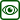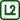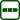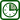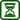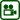## Coil in a Uniform Magnetic Field

### Experiment number : 1776

• #### Goal of experiment

This experiment shows an unusual way of generating induced voltage – by deforming a coil in a homogenous magnetic field.

The experiment is based on the research which found out that students often do not perceive that deformation of a coil near a magnet induces voltage in the coil. Conversely, if the coil rotates about an axis perpendicular to the surface of the magnet, the students often believe that voltage is induced.

This experiment is therefore meant for better understanding the concept of magnetic flux and for showing what the surface area in the definition magnetic flux is.

• #### Theory

If a coil is placed in a magnetic field, electric voltage is induced in the coil provided that the magnetic flux changes. Magnetic flux describes the density of magnetic field lines entering perpendicularly the surface area S (in our case the surface area of the coil)

$\Phi=B\cdot S$

If the magnetic field lines do not enter the surface area perpendicularly, but the angle between them and normal of the surface area is α, the magnetic flux is given by the relation:

$\Phi=B\cdot S \cos\alpha \tag{1}$

The unit of magnetic flux is Weber.

The voltage which is induced in a coil is determined by Faraday's law of electromagnetic induction:

$U_i=-N\frac{\Delta\Phi}{\Delta t},\tag{2}$ where N is the number of turns of the coil.

From the relation (2) follows that the more we change the magnetic flux, the greater the induced voltage is. The change of magnetic flux and thus the generation of induced voltage can be arranged in several ways, as can be seen from equation (1):

1. Changing the magnitude of magnetic field
2. Changing the surface area of the coil
3. Changing the angle between the surface area and the magnetic field lines.

At school mainly the first method can be encountered – a magnet is pushed into the coil and pulled out, thus changing the magnetic field. In power plants, electricity is generated by an (electro)magnet rotating between three coils, which changes not only the magnitude of electric field, but also the angle between the coil and magnetic field lines.

The second way of changing the magnetic field is not met very often, because coils that are used in schools are not easily deformed.

• #### Tools

• plate with magnets glued to it
• coil with approx. 20 turns wound from a flexible wire
• voltmeter
• conductors, crocodile clips
• laminated I core
• #### The plate with magnets and the coil

The main tool is a plate on which flat 2 x 4 cm and 1 mm thick neodymium magnets (see Fig. 1) are glued. The magnets are glued with the same poles facing upwards and with very small gaps between the magnets. Thus, the magnetic field near the plate is homogeneous.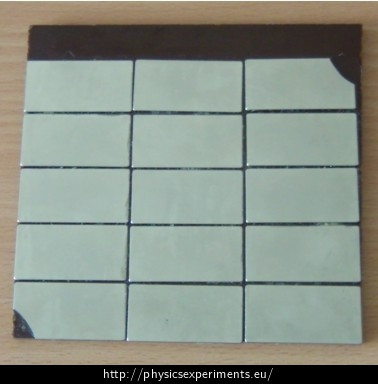The second essential tool is a coil with about twenty turns (see Fig. 2). The coil is wound from a soft wire, so that it can be deformed.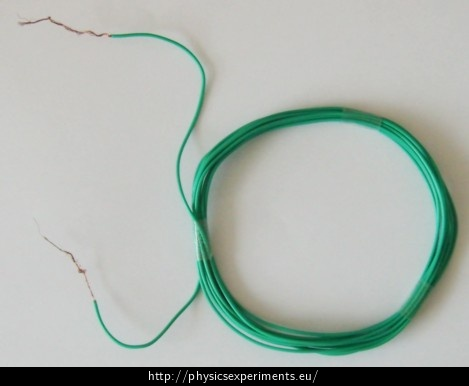• #### Procedure

Connect the coil to the voltmeter and place it on the plate with magnets (see Fig. 3).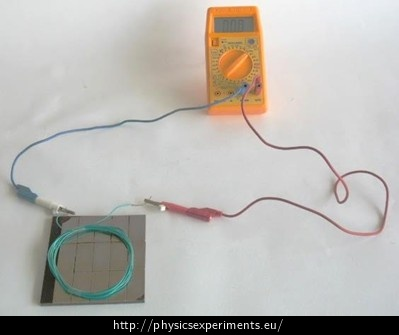Verify that voltage is induced in the coil when:

• we deform the coil and thus change its surface area
• we move the coil up and down (we have to be sufficiently far from the plate so that the filed is no longer homogenous)
• we push the coil out of the plate (which changes the surface area through which the magnetic field lines pass
• we rotate the coil about horizontal axis thus changing the angle between the surface area of the coil and the magnetic field lines.

Conversely, verify that voltage is not induced when:

• the coil is at rest (the magnetic flux flows through the coil, but it does not change)
• we rotate the coil about vertical axis (given the fact that the field is basically homogenous, the magnetic flux does not change)
• we put the I core inside the coil and then deform the coil. In this case, most of the magnetic field lines pass through the core, which means that the important ‘surface area’ is the base of the I core; the rest of the area has a negligible effect.
• #### Sample result

The video showing a sample result is divided into two parts:

1. Voltage is induced in the coil:

2. Voltage is not induced in the coil: